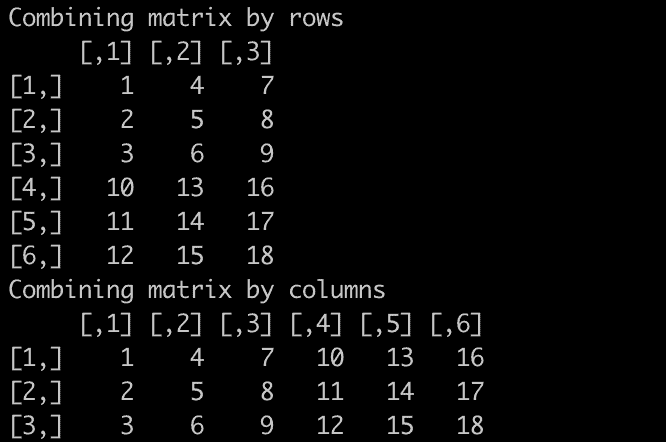# R Matrix: Create, Access, Edit, and Delete Matrix

R matrix is a two-dimensional, rectangular data structure that consists of elements of the same atomic type (most commonly numeric, but can be logical, character, or complex).

Matrices are suited for mathematical and statistical operations, where linear algebra (like matrix multiplication, transpose, etc.) plays a key role.

## Creating a Matrix

To create a matrix in R, you can use the matrix() function. The nrow and ncol properties define the dimension of the Matrix.

### Syntax

``matrix(values, nrow=3, ncol=3)``

### Parameters

1. values: The first parameter values are required, containing the values that must be filled in the Matrix.
2. nrow and ncol: The nrow and ncol parameters can be optional if one is provided. For example, if your Matrix’s length is 9 and if you give the nrow =3 then you don’t have to provide ncol. It will take ncol = 3 automatically.

### Return value

It returns the Matrix of provided values and dimensions.

### Example

``````vec <- 1:9

mtrx <- matrix(vec, 3, 3)

mtrx
``````

You will see the following (3 * 3) matrix.

``````    [,1] [,2]   [,3]
[1,]  1    4     7
[2,]  2    5     8
[3,]  3    6     9``````

It is not necessary to provide both dimension values. However, if one of the values of the dimension is provided, the other will take from the length of the data.

``````mtrx <- matrix(1:9, nrow = 3)

print(mtrx)``````

Output

``````    [,1] [,2] [,3]
[1,] 1    4    7
[2,] 2    5    8
[3,] 3    6    9``````

We can change this order and fill the matrix row-wise.

``````vec <- 1:9

mtrx <- matrix(vec, nrow = 3, byrow = TRUE)

print(mtrx) ``````

Output

``````    [,1][,2][,3]
[1,]  1   2   3
[2,]  4   5   6
[3,]  7   8   9``````

## Assigning columnnames and rownames in Matrix

To assign columnnames and rownames, use the dimnames property. For example, we can name the columns and rows by passing a two-element list to the argument dimnames.

``````vec <- 1:9

mtrx <- matrix(vec,
nrow = 3, ncol = 3,
dimnames = list(c("R1", "R2", "R3"), c("C1", "C2", "C3"))
)

mtrx
``````

Output

``````   C1 C2 C3
R1  1  4  7
R2  2  5  8
R3  3  6  9
``````

Use the rownames(), colnames(), and column names in Matrix to access row names.

``````vec <- 1:9

mtrx <- matrix(vec,
nrow = 3, ncol = 3,
dimnames = list(c("R1", "R2", "R3"), c("C1", "C2", "C3"))
)

print(colnames(mtrx))
print(rownames(mtrx))
``````

Output

`````` "C1" "C2" "C3"
 "R1" "R2" "R3"
``````

To change the column names and row names, we can reassign the values using the following syntax.

``````colnames(x) <- c("C1","C2","C3")
rownames(x) <- c("R1","R2","R3")``````

See the following example.

``````vec <- 1:9

mtrx <- matrix(vec,
nrow = 3, ncol = 3,
dimnames = list(c("R1", "R2", "R3"), c("C1", "C2", "C3"))
)

cat("Before changing the names of row and column\n")

print(colnames(mtrx))
print(rownames(mtrx))

cat("After changing the names of row and column\n")
colnames(mtrx) <- c("K1", "K2", "K3")
rownames(mtrx) <- c("B1", "B2", "B3")

print(colnames(mtrx))
print(rownames(mtrx))
``````

#### Output

``````Before changing the names of row and column
 "C1" "C2" "C3"
 "R1" "R2" "R3"

After changing the names of row and column
 "K1" "K2" "K3"
 "B1" "B2" "B3"
``````

## Creating Matrix using cbind() and rbind()

You can also create a matrix by using the two functions cbind() and rbind(), as in column bind and row bind.

``````matrix_column_binded <- cbind(1:3, 4:6)
cat("Column-binded Matrix\n")
print(matrix_column_binded)

matrix_row_binded <- rbind(1:3, 4:6)
cat("Row-binded Matrix\n")
print(matrix_row_binded)``````

Output

``````Column-binded Matrix
[,1]   [,2]
[1,]  1      4
[2,]  2      5
[3,]  3      6

Row-binded Matrix
[,1]  [,2]   [,3]
[1,]   1     2      3
[2,]   4     5      6
``````

## Accessing elements of a matrix

You can access the elements of the matrix by using [ ] brackets. The first “1” in the bracket specifies the row position, while the second “2” specifies the column position.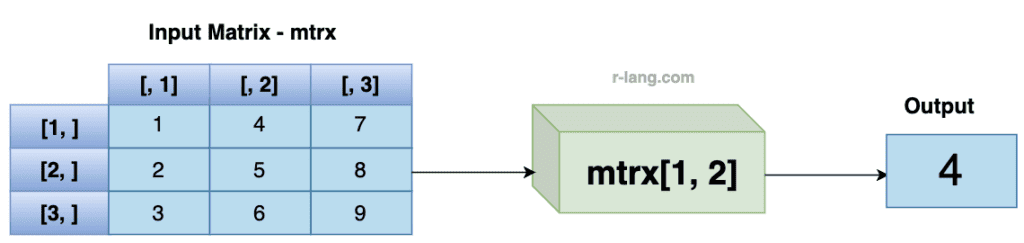``````vec <- 1:9

mtrx <- matrix(vec, nrow = 3, ncol = 3)

mtrx[1, 2]
``````

Output

`` 4``

You can also access the whole row by specifying a comma after the number in the bracket: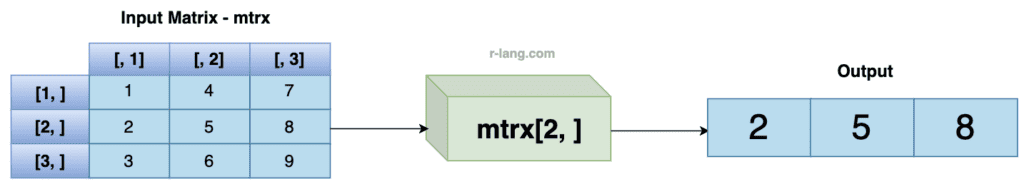``````vec <- 1:9

mtrx <- matrix(vec, nrow = 3, ncol = 3)

mtrx[2, ]
``````

Output

`` 2 5 8``

## Accessing more than one row

You can access more than one row using the c() function.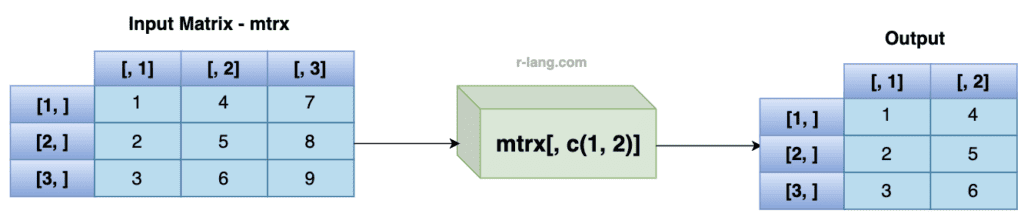``````vec <- 1:9

mtrx <- matrix(vec, nrow = 3, ncol = 3)

mtrx[, c(1, 2)]``````

Output

``````     [,1] [,2]
[1,]   1    4
[2,]   2    5
[3,]   3    6``````

## Adding a column to the matrix

You can use the cbind() function to add new columns to the matrix.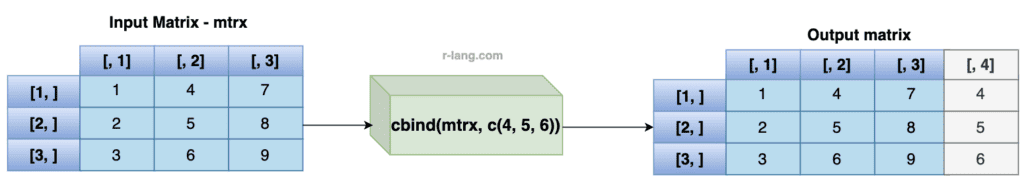``````vec <- 1:9

mtrx <- matrix(vec, nrow = 3, ncol = 3)

new_mtrx <- cbind(mtrx, c(4, 5, 6))

new_mtrx
``````

Output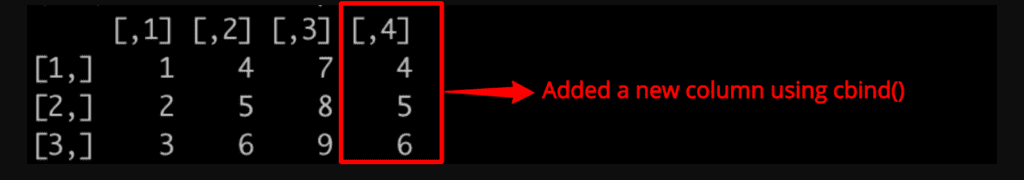## Adding a row to the matrix

You can use the rbind() function to add new rows in a Matrix.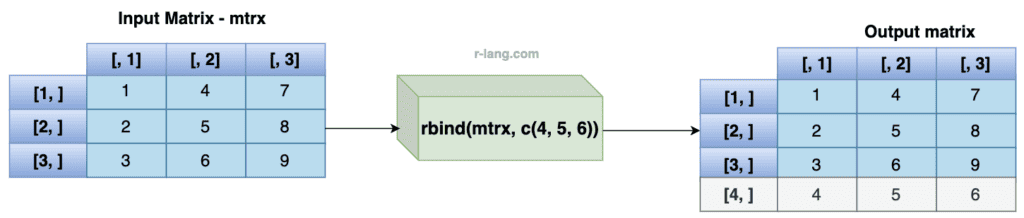``````vec <- 1:9

mtrx <- matrix(vec, nrow = 3, ncol = 3)

new_mtrx <- rbind(mtrx, c(4, 5, 6))

new_mtrx``````

Output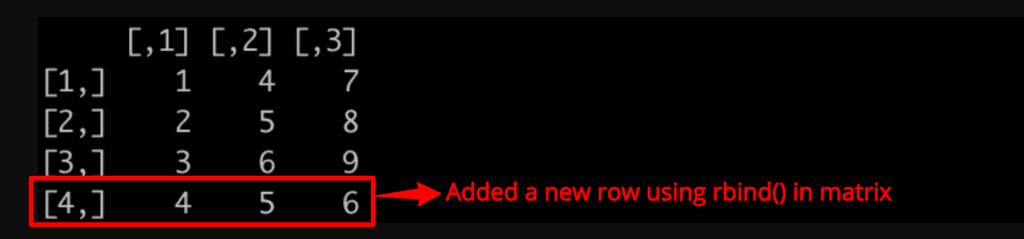## Removing columns and rows

You can use the c() function to remove rows and columns from a matrix.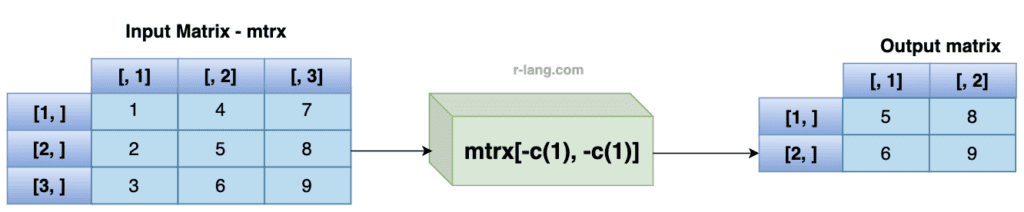Let’s remove the first row and first column from the matrix.

``````vec <- 1:9

mtrx <- matrix(vec, nrow = 3, ncol = 3)
cat("Before removing first column and row", "\n")
mtrx

cat("After removing first column and row", "\n")
new_mtrx <- mtrx[-c(1), -c(1)]
new_mtrx
``````

Output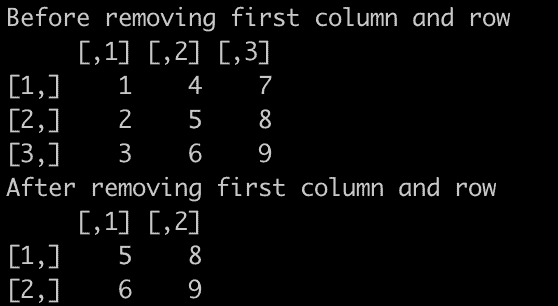## Check if an element exists in a matrix

To check if an element exists in a matrix, use the %in% operator”.

The %in operator% returns TRUE if an element exists and if it does not then it returns FALSE.

``````vec <- 1:9

mtrx <- matrix(vec, nrow = 3, ncol = 3)

5 %in% mtrx``````

Output

`````` TRUE
``````

Let’s write an example where an element does not exist in a matrix.

``````vec <- 1:9

mtrx <- matrix(vec, nrow = 3, ncol = 3)

11 %in% mtrx
``````

Output

`````` FALSE
``````

## Matrix length

To find the length of a matrix, use the “length()” function.

``````vec <- 1:9

mtrx <- matrix(vec, nrow = 3, ncol = 3)

length(mtrx)``````

Output

`` 9``

## Finding the Number of Rows and Columns of the Matrix

You can use the “dim()” function to find the number of rows and columns in a Matrix.

``````vec <- 1:9

mtrx <- matrix(vec, nrow = 3, ncol = 3)

dim(mtrx)``````

Output

``````  3   3
``````

## Combining two matrices using rbind() and cbind()

Use the rbind() or cbind() functions to combine two or more matrices together:

``````vec1 <- 1:9
vec2 <- 10:18

mtrx1 <- matrix(vec1, nrow = 3, ncol = 3)
mtrx2 <- matrix(vec2, nrow = 3, ncol = 3)

# Adding it as a rows
combined_matrix_by_rows <- rbind(mtrx1, mtrx2)
cat("Combining matrix by rows", "\n")
combined_matrix_by_rows

# Adding it as a columns
combined_matrix_by_cols <- cbind(mtrx1, mtrx2)
cat("Combining matrix by columns", "\n")
combined_matrix_by_cols``````

Output